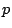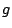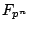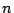# Computing the local factors of higher genus curve

Not much is known about computing zeta functions of curves of genus greater than 1; all known methods involve computing-adic approximations of the zeta function, so are limited to small characteristic. Attempts to generalize the methods of the previous section have mostly been unsuccessful. A generalization ``in principle'' of Schoof's algorithm to higher genus was given by Pila [ MR 91a:11071], but it requires explicit equations for the Jacobian of the curve. It is possible this could be carried out in genus 2, but seems hopeless for higher genus. Satoh's method hinges on the existence of the canonical lift; in higher genus, the Jacobian of an ordinary curve admits a Serre-Tate lift as a principally polarized abelian variety, but the lift need not be a Jacobian, making working with it difficult. In genus 2, this problem does not arise, and Harley (Midwest Algebraic Geometry in Cryptography 2001 talk) has proposed a genus 2 version of the AGM method that will probably be quite efficient in practice.

A very general algorithm of Lauder and Wan [A.G.B. Lauder and D. Wan, Counting points on varieties over finite fields of small characteristic, preprint], based on Dwork's proof of the rationality of the zeta function, gives an algorithm for computing the zeta function of a genuscurve overin time polynomial in,and. However, the algorithm seems difficult to implement in practice. Lauder and Wan also have a simplified version [A.G.B. Lauder and D. Wan, Computing zeta functions of Artin-Schreier curves over finite fields, preprint] of their algorithm in the special case of Artin-Schreier curves of-rank 0, and it is likely that other special cases can be handled efficiently.

A related distinct approach is used in an algorithm of Kedlaya [ arXiv:math.AG/0105031](K.S. Kedlaya, Counting points on hyperelliptic curves using Monsky-Washnitzer cohomology, J. Ramanujan Math. Soc. 16,(2001), 323-338), which computes the zeta function of a genushyperelliptic curve over(odd) in time polynomial in,and. This algorithm uses the Lefschetz trace formula for Monsky-Washnitzer cohomology. This technique has been extended by Gaudry and Gurel [An extension of Kedlaya's algorithm to superelliptic curves, to appear in Advances in Cryptology--ASIACRYPT 2001, Springer-Verlag Lecture Notes in Computer Science] to handle superelliptic curves, and by Denef and Vercauteren [J. Denef and F. Vercauteren, An extension of Kedlaya's algorithm to Artin-Schreier curves in characteristic 2, preprint] to handle Artin-Schreier curves of-rank 0 or 1; again, it is likely that other cases can be handled efficiently. (Denef and Vercauteren, in their paper, indicate that they can handle general Artin-Schreier curves, and in fact arbitrary curves, but details are not yet available.)

Remaining questions include:

• developing efficient implementations of the above algorithms;
• developing algorithms for more general curves;
• developing algorithms for higher dimensional varieties.
Once efficient implementations are available, it will be possible to conduct some new experiments, e.g., determine experimentally the distribution of the zeroes of the zeta function over all hyperelliptic curves of a given large genus over a given small field.

Back to the main index for L-functions and Random Matrix Theory.